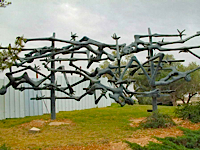N O P Q R S T U V W X Y Z

A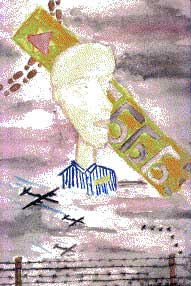N O P Q R S T U V W X Y Z

B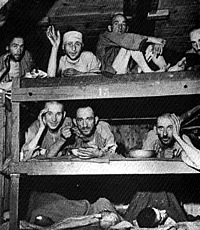N O P Q R S T U V W X Y Z

CN O P Q R S T U V W X Y Z

DN O P Q R S T U V W X Y Z

EN O P Q R S T U V W X Y Z

FN O P Q R S T U V W X Y Z

GN O P Q R S T U V W X Y Z

HN O P Q R S T U V W X Y Z

IN O P Q R S T U V W X Y Z

KN O P Q R S T U V W X Y Z

LN O P Q R S T U V W X Y Z

MN O P Q R S T U V W X Y Z

NN O P Q R S T U V W X Y Z

ON O P Q R S T U V W X Y Z

P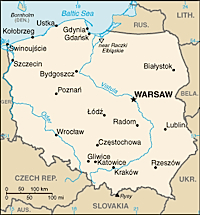N O P Q R S T U V W X Y Z

RN O P Q R S T U V W X Y Z

SN O P Q R S T U V W X Y Z

TN O P Q R S T U V W X Y Z

VN O P Q R S T U V W X Y Z

W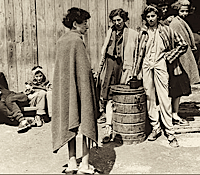N O P Q R S T U V W X Y Z

Y